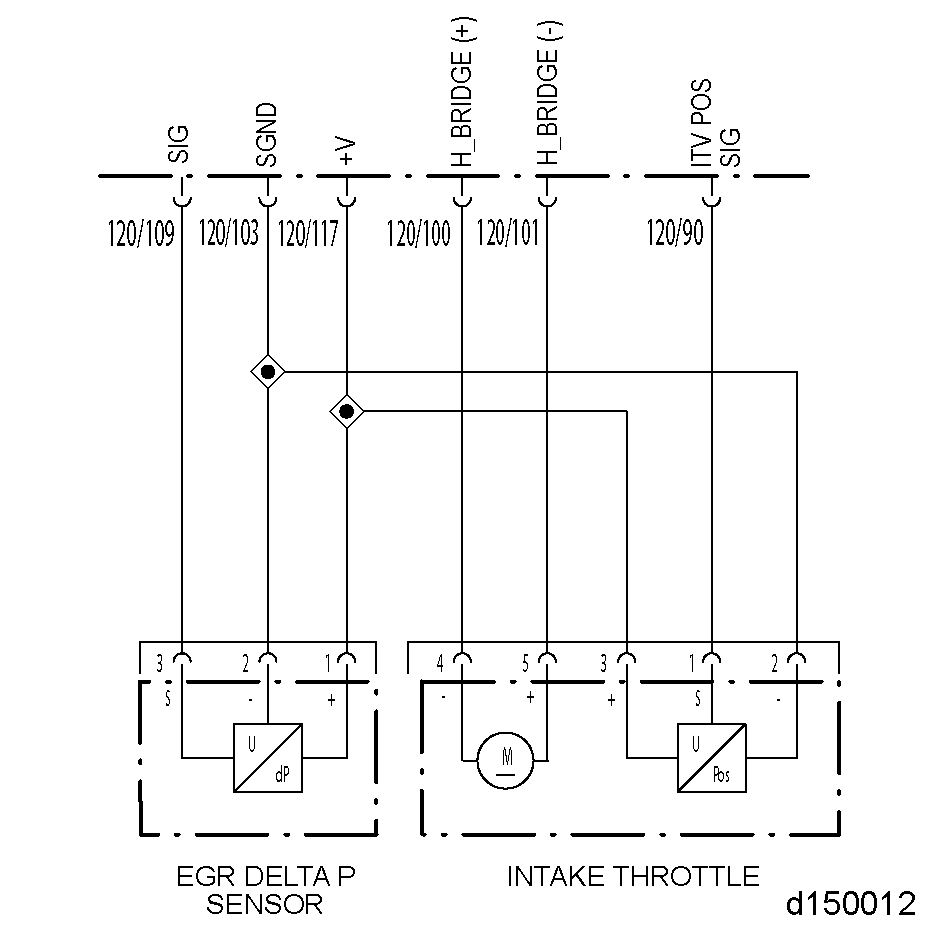# Section 39.4 SPN 411/FMI 4

### Section 39.4 SPN 411/FMI 4

This diagnosis is typically EGR Delta P Sensor short to ground.‪Check as follows:‪

1. Disconnect the EGR Delta P Sensor.
2. Disconnect the 120–pin MCM connector.
3. Measure the resistance between pins 1 and 2 of the EGR Delta P Sensor.
1. If the resistance is greater than 5Ω, go to the next step.
2. If the resistance is less than 5Ω, repair the short between pins 103 and 117 of the 120–pin MCM connector. Refer to "39.4.1 Verify Repairs" .
4. Measure the resistance between pins 2 and 3 of the EGR Delta P Sensor.
1. If the resistance is greater than 5Ω, go to the next step.
2. If the resistance is less than 5Ω, repair the short between pins 103 and 109 of the 120–pin MCM connector. Refer to "39.4.1 Verify Repairs" .
5. Measure the resistance between pin 1 of the EGR Delta P Sensor and ground.
1. If the resistance is greater than 3Ω, go to the next step.
2. If the resistance is less than 3Ω, repair the short circuit between pin 1 of the EGR Delta P Sensor harness connector and ground. Refer to "39.4.1 Verify Repairs" .
6. Measure the resistance between pin 3 of the EGR Delta P Sensor and ground.
1. If the resistance is greater than 3Ω, repeat the previous steps. If the results are the same, call the Detroit Diesel Customer Support Center (313–592–5800).
2. If the resistance is less than 3Ω, repair the short circuit between pin 3 of the EGR Delta P Sensor harness connector and ground. Refer to "39.4.1 Verify Repairs" .

#### Section 39.4.1 Verify Repairs

Verify repairs as follows:‪

1. Turn ignition OFF.
2. Reconnect any electrical connections that were disconnected to perform the diagnosis.ENGINE EXHAUST ‪ To avoid injury from inhaling engine exhaust, always operate the engine in a well-ventilated area. Engine exhaust is toxic.‪